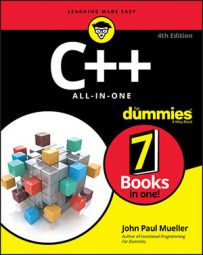##### C++ All-in-One For DummiesThe usual way of declaring an array is to simply line up the type name, followed by a variable name, followed by a size in brackets, as in this line of code:

`int Numbers;`

This code declares an array of 10 integers. The first element gets index 0, and the final element gets index 9. Always remember that in C++ arrays start at 0, and the highest index is one less than the size. (Remember, index refers to the position within the array, and size refers to the number of elements in the array.)

A common question that the usual programming student asks is, “Can I just declare an array without specifying a size?” The line would look like this:

`int Numbers[]`

In certain situations, you can declare an array without putting a number in the brackets. For example, you can initialize an array without specifying the number of elements:

`    int MyNumbers[] = {1,2,3,4,5,6,7,8,9,10};`

The compiler is smart enough to count how many elements you put inside the braces, and then the compiler makes that count the array size.

Specifying the array size helps decrease your chances of having bugs, bugs, everywhere bugs. Plus, it has the added benefit that, in the actual declaration, if the number in brackets does not match the number of elements inside braces, the compiler issues an error, at least if the number is smaller anyway. The following

`int MyNumbers = {1,2,3,4,5,6,7,8,9,10};`

yields this compiler error:

`error: too many initializers for 'int '`

But if the number in brackets is greater than the number of elements, as in the following code, you will not get an error. So be careful!

`int MyNumbers = {1,2,3,4,5,6,7,8,9,10};`

You also can skip specifying the array size when you pass an array into a function, like this:

```int AddUp(int Numbers[], int Count) {
int loop;
int sum = 0;
for (loop = 0; loop < Count; loop++) {
sum += Numbers[loop];
}
return sum;
}```

This technique is particularly powerful because the AddUp function can work for any size array. You can call the function like this:

`cout << AddUp(MyNumbers, 10) << endl;`

But this way to do it is kind of annoying because you have to specify the size each time you call in to the function. However, you can get around this problem. Look at this line of code:

`cout << AddUp(MyNumbers, sizeof(MyNumbers) / 4) << endl;`

With the array, the sizeof operator tells you how many bytes it uses. But the size of the array is usually the number of elements, not the number of bytes. So you divide the result of sizeof by 4 (the size of each element).

But now you have that magic number, 4, sitting there. (Magic number refers to a seemingly arbitrary number that’s stuffed somewhere into your code.) So a slightly better approach would be to enter this line:

`cout << AddUp(MyNumbers, sizeof(MyNumbers) / sizeof(int)) << endl;`

Now this line of code works, and here’s why: The sizeof the array divided by the sizeof each element in the array gives the number of elements in the array.